## Slicing Method

### Learning Outcomes

• Determine the volume of a solid by integrating a cross-section (the slicing method)

## Volume and the Slicing Method

Just as area is the numerical measure of a two-dimensional region, volume is the numerical measure of a three-dimensional solid. Most of us have computed volumes of solids by using basic geometric formulas. The volume of a rectangular solid, for example, can be computed by multiplying length, width, and height: $V=lwh.$ The formulas for the volume of a sphere $(V=\frac{4}{3}\pi {r}^{3}),$ a cone $(V=\frac{1}{3}\pi {r}^{2}h),$ and a pyramid $(V=\frac{1}{3}Ah)$ have also been introduced. Although some of these formulas were derived using geometry alone, all these formulas can be obtained by using integration.

We can also calculate the volume of a cylinder. Although most of us think of a cylinder as having a circular base, such as a soup can or a metal rod, in mathematics the word cylinder has a more general meaning. To discuss cylinders in this more general context, we first need to define some vocabulary.

We define the cross-section of a solid to be the intersection of a plane with the solid. A cylinder is defined as any solid that can be generated by translating a plane region along a line perpendicular to the region, called the axis of the cylinder. Thus, all cross-sections perpendicular to the axis of a cylinder are identical. The solid shown in Figure 1 is an example of a cylinder with a noncircular base. To calculate the volume of a cylinder, then, we simply multiply the area of the cross-section by the height of the cylinder: $V=A·h.$ In the case of a right circular cylinder (soup can), this becomes $V=\pi {r}^{2}h.$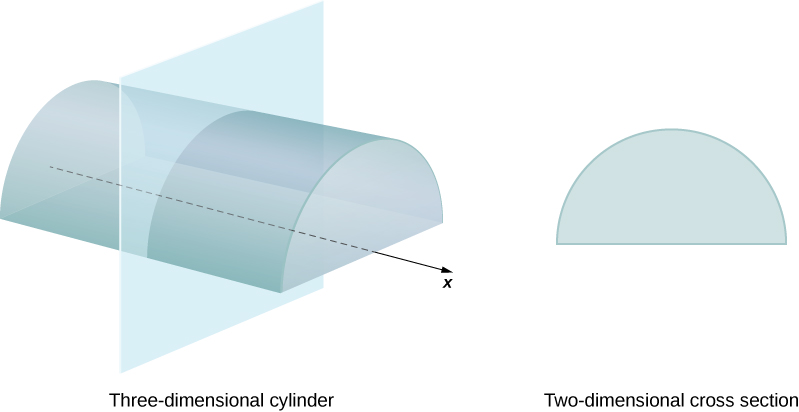Figure 1. Each cross-section of a particular cylinder is identical to the others.

If a solid does not have a constant cross-section (and it is not one of the other basic solids), we may not have a formula for its volume. In this case, we can use a definite integral to calculate the volume of the solid. We do this by slicing the solid into pieces, estimating the volume of each slice, and then adding those estimated volumes together. The slices should all be parallel to one another, and when we put all the slices together, we should get the whole solid. Consider, for example, the solid S shown in Figure 2, extending along the $x\text{-axis}\text{.}$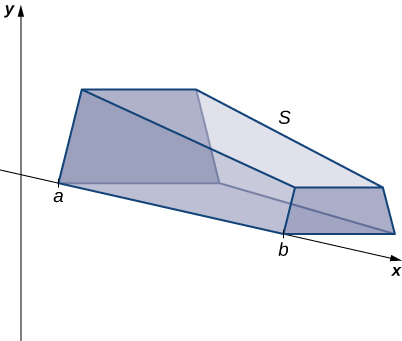Figure 2. A solid with a varying cross-section.

We want to divide $S$ into slices perpendicular to the $x\text{-axis}\text{.}$ As we see later in the chapter, there may be times when we want to slice the solid in some other direction—say, with slices perpendicular to the $y$-axis. The decision of which way to slice the solid is very important. If we make the wrong choice, the computations can get quite messy. Later in the chapter, we examine some of these situations in detail and look at how to decide which way to slice the solid. For the purposes of this section, however, we use slices perpendicular to the $x\text{-axis}\text{.}$

Because the cross-sectional area is not constant, we let $A(x)$ represent the area of the cross-section at point $x.$ Now let $P=\left\{{x}_{0},{x}_{1}\text{…},{X}_{n}\right\}$ be a regular partition of $\left[a,b\right],$ and for $i=1,2\text{,…}n,$ let ${S}_{i}$ represent the slice of $S$ stretching from ${x}_{i-1}\text{ to }{x}_{i}.$ The following figure shows the sliced solid with $n=3.$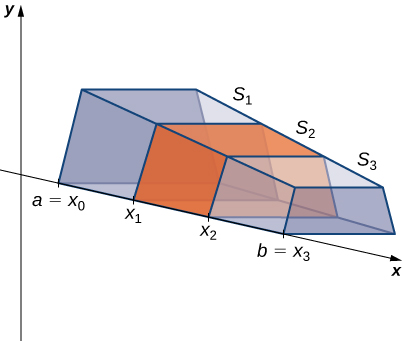Figure 3. The solid $S$ has been divided into three slices perpendicular to the $x\text{-axis}.$

Finally, for $i=1,2\text{,…}n,$ let ${x}_{i}^{*}$ be an arbitrary point in $\left[{x}_{i-1},{x}_{i}\right].$ Then the volume of slice ${S}_{i}$ can be estimated by $V({S}_{i})\approx A({x}_{i}^{*})\text{Δ}x.$ Adding these approximations together, we see the volume of the entire solid $S$ can be approximated by

$V(S)\approx \underset{i=1}{\overset{n}{\text{∑}}}A({x}_{i}^{*})\text{Δ}x$

By now, we can recognize this as a Riemann sum, and our next step is to take the limit as $n\to \infty .$ Then we have

$V(S)=\underset{n\to \infty }{\text{lim}}\underset{i=1}{\overset{n}{\text{∑}}}A({x}_{i}^{*})\text{Δ}x=\underset{a}{\overset{b}{\displaystyle\int }}A(x)dx$

The technique we have just described is called the slicing method. To apply it, we use the following strategy.

### Problem-Solving Strategy: Finding Volumes by the Slicing Method

1. Examine the solid and determine the shape of a cross-section of the solid. It is often helpful to draw a picture if one is not provided.
2. Determine a formula for the area of the cross-section.
3. Integrate the area formula over the appropriate interval to get the volume.

Recall that in this section, we assume the slices are perpendicular to the $x\text{-axis}\text{.}$ Therefore, the area formula is in terms of $x$ and the limits of integration lie on the $x\text{-axis}\text{.}$ However, the problem-solving strategy shown here is valid regardless of how we choose to slice the solid.

### Example: Deriving the Formula for the Volume of a Pyramid

We know from geometry that the formula for the volume of a pyramid is $V=\frac{1}{3}Ah.$ If the pyramid has a square base, this becomes $V=\frac{1}{3}{a}^{2}h,$ where $a$ denotes the length of one side of the base. We are going to use the slicing method to derive this formula.

### Try It

Use the slicing method to derive the formula $V=\frac{1}{3}\pi {r}^{2}h$ for the volume of a circular cone.

## Solids of Revolution and the Slicing Method

If a region in a plane is revolved around a line in that plane, the resulting solid is called a solid of revolution, as shown in the following figure.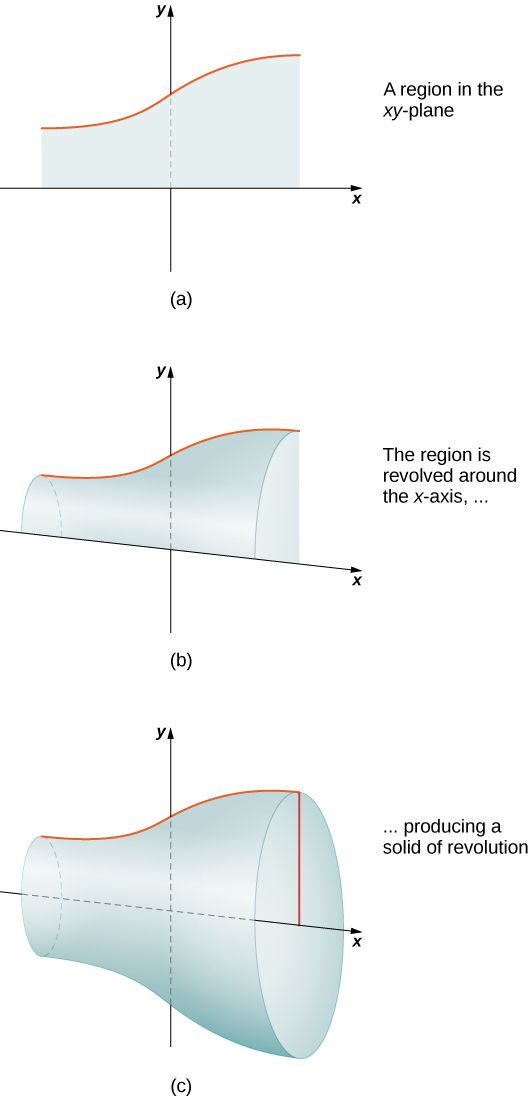Figure 5. (a) This is the region that is revolved around the x-axis. (b) As the region begins to revolve around the axis, it sweeps out a solid of revolution. (c) This is the solid that results when the revolution is complete.

Solids of revolution are common in mechanical applications, such as machine parts produced by a lathe. We spend the rest of this section looking at solids of this type. The next example uses the slicing method to calculate the volume of a solid of revolution.

### Example: Using the Slicing Method to find the Volume of a Solid of Revolution

Use the slicing method to find the volume of the solid of revolution bounded by the graphs of $f(x)={x}^{2}-4x+5,x=1,\text{ and }x=4,$ and rotated about the $x\text{-axis}\text{.}$

### Try It

Use the method of slicing to find the volume of the solid of revolution formed by revolving the region between the graph of the function $f(x)=\frac{1}{x}$ and the $x\text{-axis}$ over the interval $\left[1,2\right]$ around the $x\text{-axis}\text{.}$ See the following figure.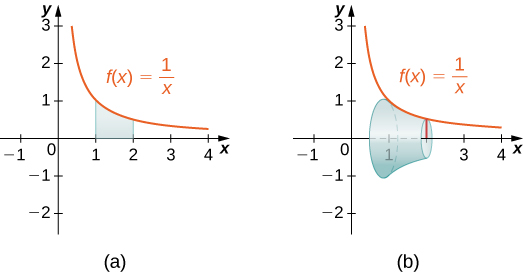Figure 8.

Watch the following video to see the worked solution to the above Try It.